# RRB PO Prelims Reasoning (Day-22)

## Reasoning Materials for RRB PO Prelims

Dear Aspirants, Our IBPS Guide team is providing new series of Reasoning Questions for RRB PO Prelims 2020 so the aspirants can practice it on a daily basis. These questions are framed by our skilled experts after understanding your needs thoroughly. Aspirants can practice these new series questions daily to familiarize with the exact exam pattern and make your preparation effective.

Start Quiz

IBPS RRB PO Prelims

Word based arrangement

Direction (1-4): Study the following information carefully and answer the below questions.

Eight alphabetical letters are arranged from left to right in such a manner to form a meaningful word. All letters are different. The word contains five consonants and three vowels.

Only two letters are between E and U. P is placed immediate left of S. The number of letters to the left of E is the same as to the right of U. The number of letters between A and P is the same as between A and C. L is placed second to the left of A. N is one of the letters in the word.

1) As many letters are between L and A is the same as between U and ____?

A.S

B.P

C.C

D.N

E.None of these

2) How many consonants are between C and U?

A.1

B.2

C.3

D.None

E.More than three

3) Which of the following statement is/are true?

A.C is placed immediate right of E

B.Three letters are between N and S

C.A and N arenot adjacent to each other

D.E is placed second to the left of N

E.All are true

4) What is the position of N with respect to S?

A.Immediate left

B.Immediate right

C.Second to the left

D.Third to the left

E.Fourth to the right

Misc

5) The Position of how many digits in the number “596472318” will remain unchanged when the digits are arranged in descending order from the left end?

A.4

B.3

C.2

D.1

E.None

Order and ranking

Direction (6-7): Study the following information carefully and answer the below questions.

There are six Bikes- X, F, G, J, Q, and Z with different weights. Bike Q is heavier than only bike X. Two bikes are in between Z and Q. Bike F is more weight than Bike G. Bike F is not the heaviest. The weight of Bike F and Bike J is 293kg and 346kg respectively.

6) How many bikes are heavier than G?

A.1

B.2

C.3

D.4

E.None

7) What may be the weight of bike Z?

A.220 kg

B.160 kg

C.334 kg

D.155 kg

E.None of these

Misc

8) If the letters in the word “AVAILABLE” are arranged in alphabetical order from left to right, then how many letters are between the letters which are fifth from the left end and fourth from the right end in the alphabetical series?

A.3

B.4

C.6

D.8

E.9

9) If in the number 271935468, the digits at the odd position are increased by 1 and the digits at the even position are decreased by 2, then how many digits thus formed are greater than six?

A.2

B.3

C.4

D.1

E.More than four

10) If a meaningful word can be formed by using T, S, H, and A using each letter only once then what is the second letter from the left end of the newly formed word? Mark Y as your answer if more than one word is formed. Mark Z if no meaningful word can be formed.

A.A

B.P

C.H

D.Y

E.Z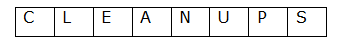Only two letters are between E and U.

The number of letters to the left of E is the same as to the right of U.

From the above condition, there are two possibilities.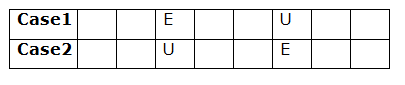L is placed second to the left of A.

From the above condition, there are four possibilities.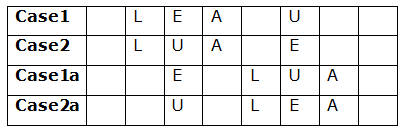P is placed immediate left of S.

The number of letters between A and P is the same as between A and C.

From the above condition, Case1a and case2a get eliminated.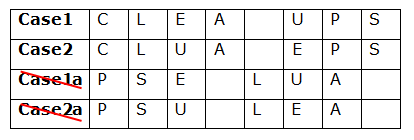N is one of the letters in the word.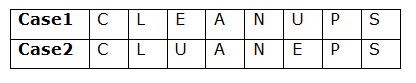From the above two cases we get CLEANUPS and CLUANEPS But among those CLEANUPS only can give a meaningful word, so it will be our answer.

So the final arrangement will be,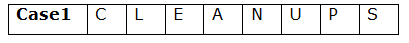596472318

987654321

J(346 kg) > Z > F (293 kg) > G > Q > X

AVAILABLE

AAABEILLV

EFGHI

271935468

352743549